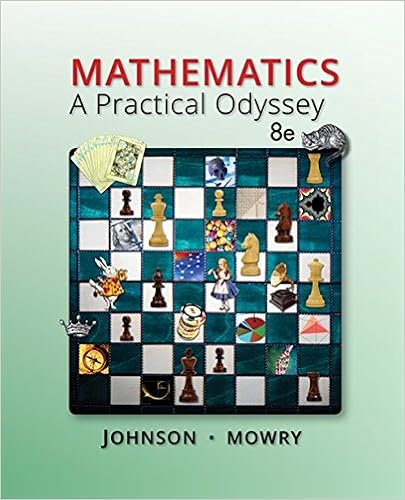# 2 determine the significance level α 3 determine the

• Notes
• 60
• 100% (1) 1 out of 1 people found this document helpful

This preview shows page 14 - 19 out of 60 pages.

##### We have textbook solutions for you!
The document you are viewing contains questions related to this textbook.The document you are viewing contains questions related to this textbook.
Chapter 1 / Exercise 5
Mathematics: A Practical Odyssey
Johnson/MowryExpert Verified
2. Determine the significance level α . 3. Determine the critical value : t α (one-sided) or t α /2 (two- sided) for tests of a population mean; z α or z α /2 for tests of a proportion. Remember that the two-sided critical values are exactly the values used for constructing a confidence interval. 4. Calculate the test statistic : the difference between the sample parameter and the test value, divided by the standard error (see next slide). 5. Reject the Null Hypothesis if the test statistic exceeds the critical value. Otherwise, Do Not Reject the Null Hypothesis .
##### We have textbook solutions for you!
The document you are viewing contains questions related to this textbook.The document you are viewing contains questions related to this textbook.
Chapter 1 / Exercise 5
Mathematics: A Practical Odyssey
Johnson/MowryExpert Verified
9-15 Hypothesis Testing and Confidence Intervals Confidence Intervals and Hypothesis Tests have most steps in common: Estimate a parameter (e.g. or p ̂ ) Find the Standard Error SE Determine the significance level α (alpha), which is complementary to the confidence level (1- α ). A low statistical significance level gives a high confidence. Look up the critical value t α /2 or z α /2 (for CI and two-sided hypothesis test) or t α or z α for one-sided test. Any two-sided hypothesis test is equivalent to a Confidence Interval test (test whether a value is included in the CI). Note that a two-sided test is more conservative than a one-sided test.
9-16 Hypothesis Testing and Confidence Intervals Any Null hypothesis can be rewritten as a test whether a parameter is zero, e. g. μ - μ 0 = 0 instead of μ = μ 0 . Then we can calculate the margin of error, construct a CI for ( μ - μ 0 ) and test whether it includes zero, in other words, whether the distance | - μ 0 | is greater than the CI margin of error, E= t α /2 SE . The Null Hypothesis always represents the absence of the effect being tested. The distance | - μ 0 | is a measure of the size of the observed effect. For an effect to be significant, it has to be large in measures of the standard error. Therefore we use the test statistic ( - μ 0 )/SE and compare it to the critical value t α /2 . This is exactly equivalent to the CI approach except in case of a one-sided test, when we use t α as critical value.
9-17 Hypothesis Testing Important Note !!! There are two and only two possible outcomes of a statistical Hypothesis test: Reject the Null Hypothesis, OR Do Not Reject the Null Hypothesis . !!! You must be able to interpret what it means to reject or not reject the Null Hypothesis. Reject H 0 : there is strong evidence in favor of H a Do Not Reject H 0 : there is insufficient evidence in favor of H a
9-18 Understanding Hypothesis Testing Keep in mind these general caveats: Any statistically significant effect could still be due to chance. Even a small effect could be statistically significant. Conversely, a large effect might not appear statistically significant if the sample size is too small. Statistical significance is not the same as "real world" significance!
•••